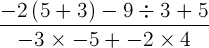# KCSE Mathematics Past Papers

Find KCSE Mathematics Paper 1 2010. Kenya Certificate of Secondary Education Mathematics Paper 1 Past paper

## KCSE Mathematics Paper 1 2010

SECTION A (50 MARKS)

1. Without using a calculator evaluate:(3 marks)

2. Kutu withdrew some money from a bank. He spent 3/8 of the money to pay for Mutua’s school fees and 2/5 to pay for Tatu’s school fees. If he remained with Ksh 12 330, calculate the amount of money he paid for Tatu’s school fees.     (4 marks)

3. A straight line L passes through the point (3, -2) and Is perpendicular to a line whose equation is 2y – 4x = 1.

Find the equation of L in the form y = mx + c, where m and c are constants.      (3 marks)

4. A bus left a petrol station at 9.20a.m. and traveled at an average speed of 75km/h to a town N. At 9.40 a.m. a taxi. traveling at an average speed of 95km/h, left the same petrol station and followed the route of the bus. Determine the distance, from the petrol station, covered by the taxi at the time it caught up with the bus.         (3 marks)

5. The sum of three consecutive odd integers is greater than 219. Determine the the first three such integers.      (3 marks)

6. A Kenyan company received US Dollars 100 000. The money was converted into Kenya shillings in a bank which buys and sells foreign currencies as follows:

 Buying (in Kenya shillings) Selling (in Kenya shillings) 1 US Dollar 77.24 77.44 1 Sterling Pound 121.93 122.27

(a) Calculate the amount of money, in Kenya shillings, the company received.      (2 marks)

(b) The company exchanged the Kenya shillings calculated in (a) above, into sterling pounds to buy a car from Britain. Calculate the cost of the car to the nearest sterling pound.      (2 marks)

7. In the figure below, OPQR is a trapezium in which PQ is parallel to OR and M is the mid-point of QR. OP = p, OR = r and PQ = 1/3OR.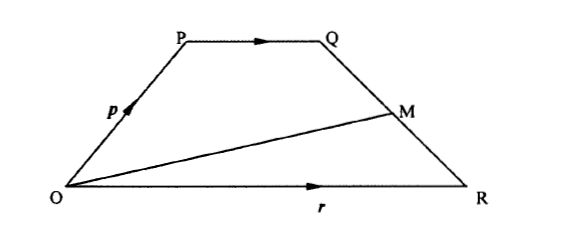Find OM in terms of p and r                            (3 marks)

8. Without using mathematical tables or a calculator, evaluate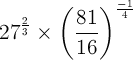(3 marks)

9. The figure below is a net of a cube with some dots on two faces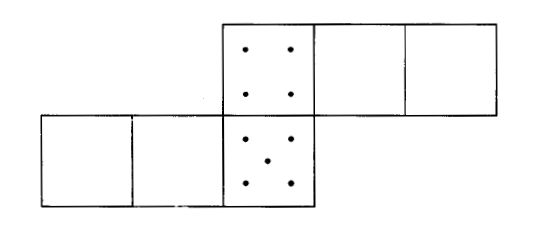Given that the number of dots on pairs of opposite faces add up to 7, fill in appropriate dots in each of the empty faces.       (2 marks)

10. Using a ruler and a pair of compasses only, construct a rhombus QRST in which angle TQR=60°and QS=10cm.        (3 marks)

11. A fruit vendor bought 1948 oranges on a Thursday and sold 750 of them on the same day. On Friday, he sold 240 more oranges than on Thursday. On Saturday he bought 560 more oranges. Later that day, he sold all the oranges he had at a price of Ksh 8 each. Calculate the amount of money the vendor obtained from the sales of Saturday.      (4 marks)

12.  Simplify the expression: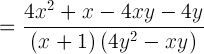(3 marks)

13. Given that 3Ɵ = Cos 2Ɵ° find the value of Ɵ.

14.  A cylindrical solid whose radius and height are equal has a surface area of 154cm2. Calculate its diameter, correct to 2 decimal places. (Take pi  = 3.142)       (3 marks)

15. The figure below shows two sectors in which CD and EF are arcs of concentric circles, centre 0. Angle COD = 2/3 radians and CE = DF = 5cm.If the perimeter of the shape CDFE is 24cm, calculate the length of OC.      (3 marks)

16. The histogram shown represents the distribution of heights of seedlings of a certain plant.The shaded area in the histogram represents 20 seedlings. Calculate the percentage number of seedlings with heights of at least 23cm but less than 27cm.            (3 marks)

17. A saleswoman is paid a commission of 2% on goods sold worth over Ksh 100 000. She is also paid a monthly salary of Ksh 12 000. In a certain month, she sold 360 handbags at Ksh 500 each.

(a) Calculate the saleswoman’s earnings that month.     (3 marks)

(b) The following month, the saleswoman’s monthly salary was increased by 10%. Her total earnings that month were Ksh 17 600.

Calculate:

(i) the total amount of money received from the sales of handbags that month;      (5 marks)

(ii) the number of handbags sold that month.         (2 marks)

18. A carpenter constructed a closed wooden box with internal measurements 1.5 metres long, 0.8 metres wide and 0.4 metres high. The wood used in constructing the box was 1.0 cm thick and had a density of 0.6g/cm3.

(a) Determine the:

(i) volume, in cm3, of the wood used in constructing the box;     (4 marks)

(ii) mass of the box, in kilograms, correct to 1 decimal place.      (2 marks)

(b) Identical cylindrical tins of diameter 10cm, height 20cm with a mass of 120g each were packed in the box.

Calculate the:

(i) maximum number of tins that were packed;     (2 marks)

(ii) total mass of the box with the tins.     (2 marks)

19. (a) Find A-1, the inverse of matrix: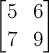(2 marks)

(b) Okello bought 5 Physics books and 6 Mathematics books for a total of Ksh 2,440. Ali bought 7 Physics books and 9 Mathematics books for a total of Ksh 3,560.

(i) Form a matrix equation to represent the above information.    (1 mark)

(ii) Use matrix method to find the price of a Physics book and that of a Mathematics book.     (3 marks)

(c) A school bought 36 Physics books and 50 Mathematics books. A discount of 5% was allowed on each Physics book whereas a discount of 8% was allowed on each Mathematics book. Calculate the percentage discount on the cost of all the books bought.    (4 marks)

20. The boundaries PQ, QR, RS and SP of a ranch are straight lines such that: Q is 16km on a bearing of 040° from P, R is directly south of Q and east of P and S is 12km on a bearing of 120° from R.

(a) Using a scale of 1cm to represent 2km, show the above information in a scale drawing.     (3 marks)

(b) From the scale drawing determine:

(i) the distance, in kilometers, of P from S           (2 marks)

(ii) the bearing bf P from S            (2 marks)

(d) Calculate the area of the ranch PQRS in square kilometres.     (3 marks)

21.  Motorbike A travels at 10km/h faster than motorbike B whose speed is x km/h. Motorbike A takes 11/2 hours less than motorbike B to cover a 180 km journey.

(a) Write an expression in terms of x for the time taken to cover the 180km Journey by:

(i) motorbike A         (1 mark)

(ii) motorbike B        (1 mark)

(b) Use the expression in (a) above to determine the speed, in km/h of motorbike A.             (6 marks)

(c) For a journey of 48km, motorbike B starts 10 minutes ahead of motorbike A. Calculate, in minutes, the difference in the time of their arrival at the destination.   (2 marks)

22. In the figure below, ABCD is a square. Points P, Q, R and S are the midpoints of AB, BC, CD and DA respectively.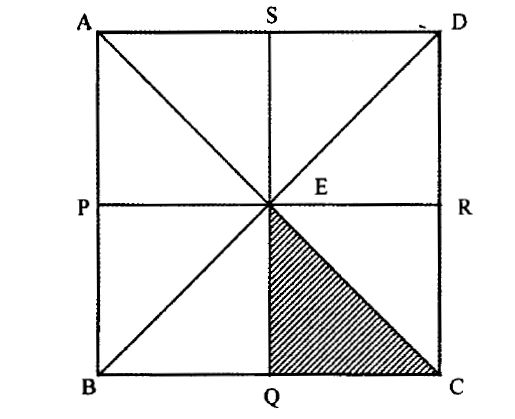(a) Describe fully:

(i) a reflection that maps triangle QCE onto triangle SDE;    (1 mark)

(ii) an enlargement that maps triangle QCE onto triangle SAE;   (2 marks)

(iii) a rotation that maps triangle QCE onto triangle SED.    (3 marks)

(b) The triangle ERC is reflected on the line BD. The image of ERC under the reflection is rotated clockwise through an angle of 90° about P. Determine the images of R and C:

(i) under the reflection;    (2 marks)

(ii)  after the two successive transformations.    (2 marks)

23. The frequency distribution table below represents the number of kilograms of meat sold in a butchery.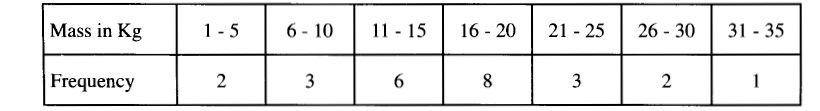(a) State the modal frequency.      (1 mark)

(b) Calculate the mean mass.     (5 marks)

(c) Calculate the median mass.     ( 4 marks)

24. A rectangular box open at the top has a square base. The internal side of the base is x cm long and the total internal surface area of the box is 432cm2.

(a) Express in terms of x:

(i) the internal height h, of the box;         (3 marks)

(ii) the internal volume V, of the box.    (1 mark)

(b) Find:

(i) the value of x for which the volume V is maximum;    (4 marks)

(ii) the maximum internal volume of the box.    (2 marks)

## KCSE Mathematics Paper 2 2010

Searching for KCSE Mathematics Past papers? Find KCSE Mathematics Paper 2 2010. Kenya Certificate of Secondary Education Mathematics Paper 2 Past paper

SECTION 1 (50 MARKS)

1. The length and width of a rectangle measured to the nearest millimeter are 7.5cm and 5.2cm respectively. Find, to four significant figures, the percentage error in the area of the rectangle.    (3 marks)

2. Simplify: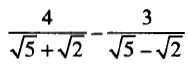3. In the figure below, 0 is the centre of the circle which passes through the points T, C and D. Line TC is parallel to OD and line ATB is a tangent to the circle at T. Angle DOC = 36°.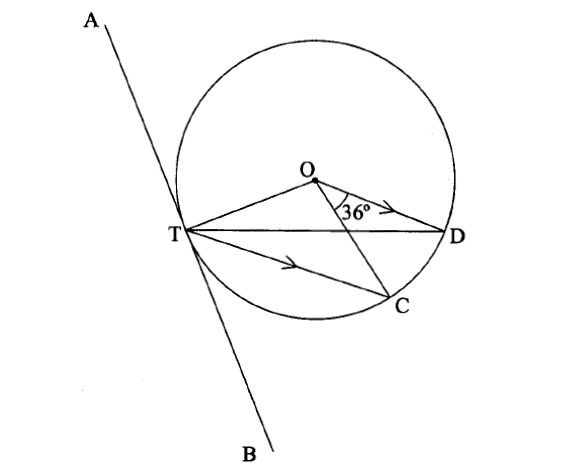Calculate the size of angle CTB.         (3 marks)

4. A tea dealer mixes two brands of tea, x and y, to obtain 35kg of the mixture worth Ksh 62 per kg. If brand x is valued at Ksh 68 per kg and brand y at Ksh 53 per kg, calculate the ratio, in its simplest form, in which the brands x and y are mixed.      (2 marks)

5. The length of a flower garden is 2m less than twice its width. The area of the garden is 60m2. Calculate its length.    (3 marks)

6. Five people can build 3 huts in 21 days. Find the number of people, working at the same rate that will build 6 similar huts in 1 5 days.        (2 marks)

7. When Ksh 40 000 was invested in a certain bank for 5 years it earned a simple interest of Ksh 3 800. Find the amount that must have been invested in the same bank at the same rate for 7 % years to earn a simple interest of Ksh 3 420.      (3 marks)

8. The heights, in centimeters. of 100 tree seedlings are shown in the table below.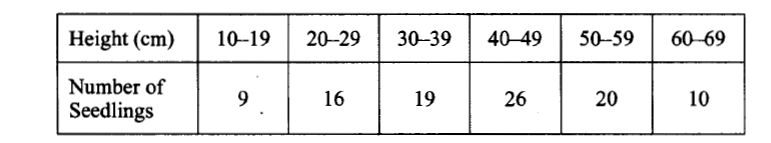Find the quartile deviation of the heights.     (4 marks)

9. A bag contains 2 white balls and 3 black balls. A second bag contains 3 whiteballs and 2 black balls. The balls are identical except for the colours. Two balls are drawn at random, one after the other from the first bag and placed in the second bag. Calculate the probability that the 2 balls are both white.      (2 marks)

10. The points 0, A and B have the coordinates (0,0) (4,0) and (3,2) respectively. Under a shear represented by the matrix triangle OAB maps onto triangle OAB’.

(a) Determine in terms of k, the x coordinate of point B’.      (2 marks)

(b) If OAB’ is a right angled triangle in which angle OB’A is acute, find two possible values of k.      (2 marks)

11. A particle starts from 0 and moves in a straight line so that its velocity V ms-1 after t seconds is given by V = 3t – t2. The distance of the particle from 0 at time t seconds is F metres.

(a) Express s in terms of t and c where c is constant.     (1 mark)

(b) Calculate the time taken before the particle returns to 0.     (3 marks)

12.(a) Expand and simplify (2 – x)5.       (2 marks)

(b) Use the first 4 terms of the expansion in part (a) above to find the approximate value of (1.8)5 to 2 decimal places.      (2 marks)

13.(a) Using line AB given below, construct the locus of a point P such that ZAPB = 90°.    (1 mark)

(b) On the same diagram locate two possible positions of point C such that point C is on the locus of P and is equidistant from A and B.        (2 marks)14. Make x the subject of the equation.

EQ

15. Find the value of x given that:  log (15 – 5x) – 1 log (3x – 2)     (3 marks)

16. The circle shown below cuts the x-axis at (-2,0) and (4,0). 4t also cuts y-axis at  (0,2) and (0,-4)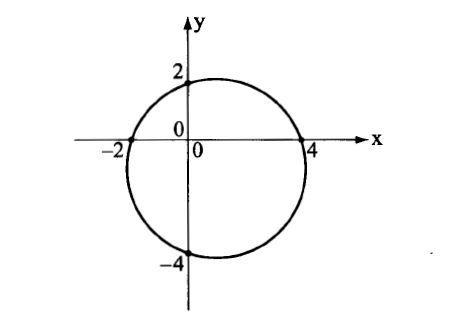Determine the:

(a) (i) coordinates of the centre     (1 mark)

(ii) radius of the circle     (1 mark)

(b) Equation of the circle in the form x2 + y2 + ax + by are constants.     (2 marks)

17.(a) Complete the table below, giving the values correct to 2 decimal places.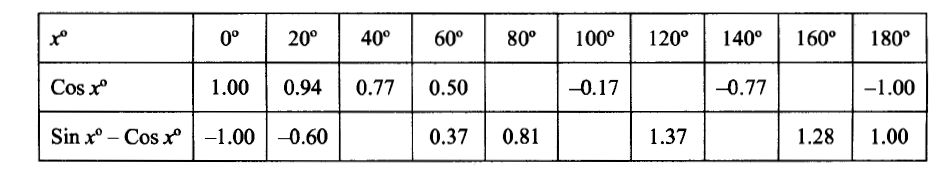(b) On the grid provided and using the same axes draw the graphs of y = coxs :x° and y = sin x° – cos x° for 0° < x < 180°. Use the scale 1 cm for 20°  on the x-axis and 4cm for 1 unit on the y-axis.     (5 marks)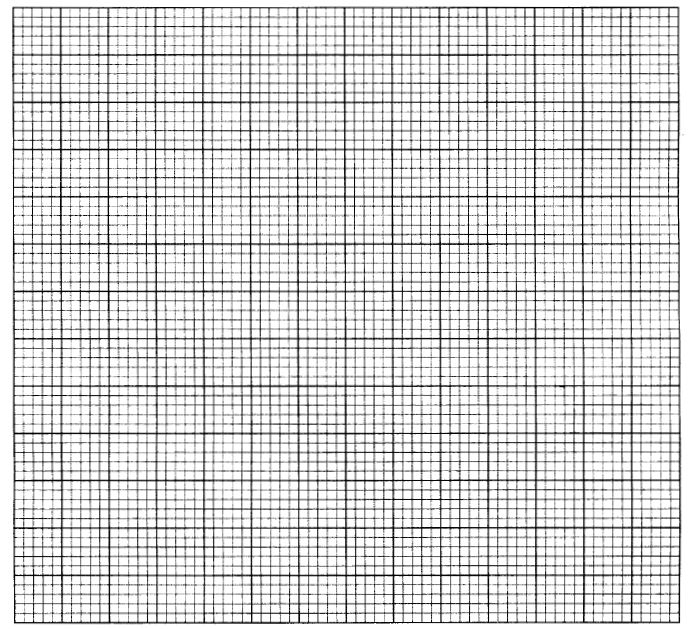(c) Using the graph in part (b):

(i) solve the equation sin x° – cos x° = 1.2     (1 mark)

(ii) solve the equation cos x0 = Vz sin x°;     (1 mark)

(iii) determine the value of cos x° in part (c) (ii) above.     (1 mark)

18. In the figure below OJKL is a parallelogram in which OJ = 3p and OL = 2r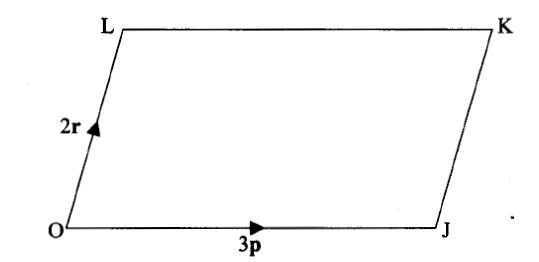(a) If A is a point on LK such that LA = 1/2AK and a point B divides the line JK externally in the ratio 3:1, express OB and AJ in terms of p and r.     (2 marks)

(b) Line OB intersects AJ at X such that OX = mOB and AX = nAJ

(i) Express OX in terms of p, r and m.                     (1 mark)

(ii) Express OX in terms of p, r and n.                     (1 mark)

(iii) Determine the values of m and n and hence the ratio in which point  X divides line AJ.       (6 marks)

19. The positions of three ports A, B and C are (34°N, 16°W), (34°N, 24°E) and (26°S, 16°W) respectively.

(a) Find the distance in nautical miles between:

(i) Ports A and B to the nearest nautical miles;           (3 marks)

(ii) Ports A and C.      (2 marks)

(b) A ship left Port A on Monday at 1330h and sailed to Port B at 40 knots.

Calculate:

(i) the local time at Port B when the ship left Port A;        (2 marks)

(ii) the day and the time the ship arrived at port B.          (3 marks)

20. A carpenter takes 4 hours to make a stool and 6 hours to make a chair. It takes  the carpenter and at least 144 hours to make x stools and y chairs.  The labour cost of making a stool is Ksh 100 and that of a chair is Ksh 200.  The total labour cost should not exceed Ksh 4 800. The carpenter must make at  least 16 stools and more than 10 chairs.

(a) Write down inequalities to represent the above information.    (3 marks)

(b) Draw the inequalities in (a) above on the grid provided.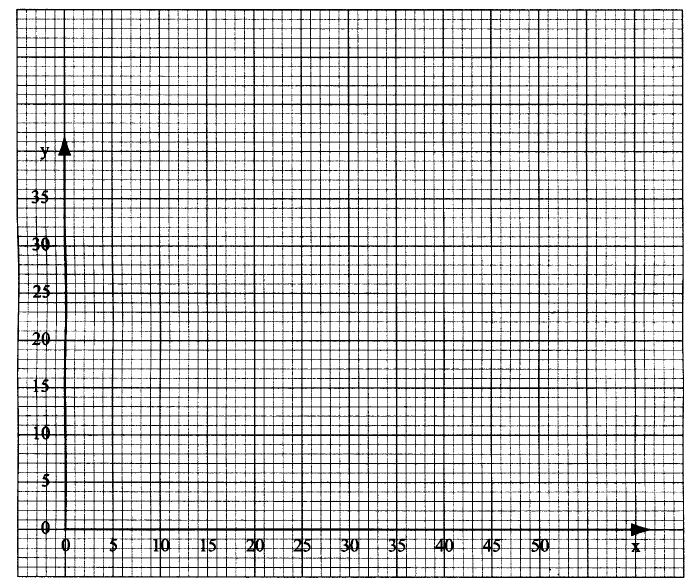(c) The carpenter makes a profit of Ksh 40 on a stool and Ksh 100 on a chair. Use the graph to determine the maximum profit the carpenter can make.     (3 marks)

21. A hall can accommodate 600 chairs arranged in rows. Each row has the same  number of chairs. The chairs are rearranged such that the number of rows are  increased by 5 but the number of chairs per row is decreased by 6.

(a) Find the original number of rows of chairs in the hall.     (6 marks)

(b) After the re-arrangement 450 people were seated in the hall leaving the same number of empty chairs in each row. Calculate the number of empty chairs per row.    (4 marks)

22. The first term of an Arithmetic Progression (A.P.) with six terms is p and its  common difference is c. Another A. P with five terms has also its first term as p  and a common difference of d. The last terms of the two Arithmetic Progressions  are equal.

(a) Express d in terms of c;      (3 marks)

(b) Given that the 4th  term of the second A.P. exceeds thp 4″1 tem of the first

one by 1 % , find the values of c and d.       (3 marks)

(c) Calculate the value of p if the sum of the terms of the first A.P is 10 more

than the sum of the terms of the second A.P.       (4 marks)

23. In a uniformity accelerated motion the distance, s metres, traveled ‘n time t seconds varies partly as the time and partly as the square of the time. When the time is 2 seconds, the distance traveled is 80 metres and when the time is 3 seconds, the distance traveled is 135 metres.

(a) Express s in terms of t.     (5 marks)

(b) Find:

(i) the distance traveled in 5 seconds.     (2 marks)

(ii) the time taken to travel a distance of 560 metres.     (3 marks)

24. In the figure below, P, Q, R and S are points on the circle centre 0. PRT and USTV are straight lines. Line USTV is a tangent to the circle at S, ZRST = 50° and ZRTV = 150°.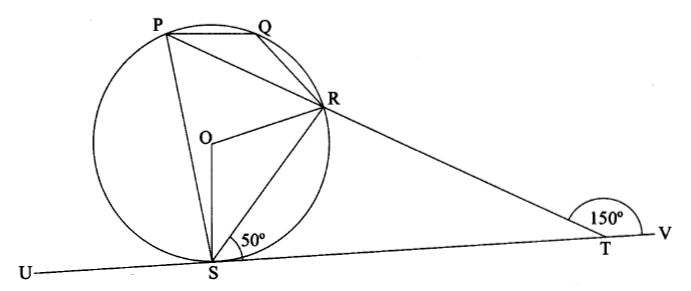(a) Calculate the size of:

(i) ORS;

(ii) USP;

(iii) PQR.

(b) Given that RT = 7cm and ST= 9cm, calculate to 3 significant figures:

(i) the length of line PR;

(ii) the radius of the circle.